# SAT Math Multiple Choice Question 942: Answer and Explanation

### Test Information

Question: 942

10. Which of the following are zeros of the quadratic equation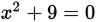? (Note: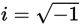)

• A.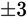• B.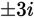• C.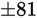• D.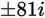Explanation:

B

Difficulty: Easy

Category: Additional Topics in Math / Imaginary Numbers

Strategic Advice: The zeros of an equation are the same as its solutions. Take a peek at the answers. The i's in some of the choices are a clue that solving by factoring may not be possible for an equation like this.

Getting to the Answer: Don't be tempted to use a factoring shortcut here-this is not a difference of two squares. The left side of the equation is a sum of two squares, so you cannot factor it. Instead, subtract 9 from both sides of the equation and use square rooting. Keep in mind that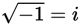: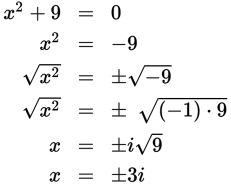This means (B) is correct.